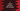# Logical operators in C with example# Logical operator in C :

Three types of logical operators are available in C. Logical AND (&&), logical OR (||) and logical NOT (!). Logical operators are used to test multiple conditions. It returns true(1) or false (0)based on the conditions. In this tutorial, I will show you these logical operators with an example for each.

## Logical OR :

Logical OR is defined using two pipe characters i.e. ||. It requires two conditions. We can use this operator to find out the logical OR of two conditions. It’s syntax is as below :

``````first_condition || second_condition
``````

It returns false only if both conditions are false. Else, the result will always true.

We don’t have true, false values in C. Instead, 1 is considered as true and 0 is considered as false i.e. it returns 0 only if both are 0.

 first_condition second_condition || result 1 0 1 0 1 1 0 0 0 1 1 1

### Example of logical OR :

``````#include <stdio.h>

int main()
{
int n = 22;

printf("%d\n", (n > 0 || (n % 2 == 0)));
printf("%d\n", (n > 0 || (n % 3 == 0)));
printf("%d\n", (n < 0 || (n % 2 == 0)));
printf("%d\n", (n < 0 || (n % 3 == 0)));
}
``````

This program will print the below output :

``````1
1
1
0
``````

In this example, we have four statements. For the first statement, both conditions are true, for the second statement, only the first condition is true, for the third statement, only the second condition is true and for the last statement, both conditions are false.

## Logical AND and NOT :

Logical AND is denoted by && and NOT is denoted by !. The result of NOT is always the opposite value. We need only one condition for NOT. AND will return true only if both values are true. For all other conditions, AND will return false.

 first_condition second_condition && result 1 0 0 0 1 0 1 1 1 0 0 0
 first_condition !result 1 0 0 1

### C example of AND:

Let’s try AND with the above example :

``````#include <stdio.h>

int main()
{
int n = 22;

printf("%d\n", (n > 0 && (n % 2 == 0)));
printf("%d\n", (n > 0 && (n % 3 == 0)));
printf("%d\n", (n < 0 && (n % 2 == 0)));
printf("%d\n", (n < 0 && (n % 3 == 0)));
}
``````

It will print :

``````1
0
0
0
``````

Only for the first statement, it returns 1 because both conditions are true.

### NOT example :

``````#include <stdio.h>

int main()
{
int n = 22;

printf("%d\n", !(n>0));
printf("%d\n", !(n<0));
}
``````

It will print :

``````0
1
``````

### Example with AND, OR and NOT :

``````#include <stdio.h>

int main()
{
int n = 22;
printf("OR : \n");
printf("%d\n", (n > 0 || (n % 2 == 0)));
printf("%d\n", (n > 0 || (n % 3 == 0)));
printf("%d\n", (n < 0 || (n % 2 == 0)));
printf("%d\n", (n < 0 || (n % 3 == 0))); printf("AND : \n"); printf("%d\n", (n > 0 && (n % 2 == 0)));
printf("%d\n", (n > 0 && (n % 3 == 0)));
printf("%d\n", (n < 0 && (n % 2 == 0)));
printf("%d\n", (n < 0 && (n % 3 == 0))); printf("NOT : \n"); printf("%d\n", !(n > 0));
printf("%d\n", !(n < 0));
}
``````

Output :

``````OR :
1
1
1
0
AND :
1
0
0
0
NOT :
0
1
``````